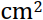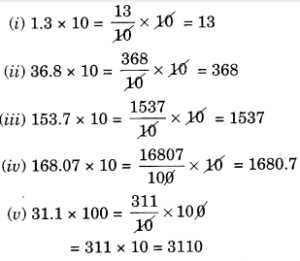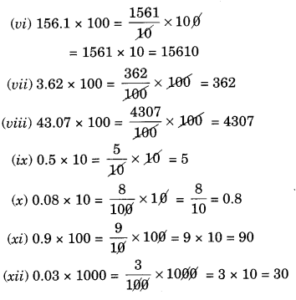# Free Ncert Solution for Class 7 Maths Chapter 2 Fractions and Decimals Exercise 2.4

Fractions and Decimals Class 7 Ex. 2.4;

New Ncert Solution for Class 7 Maths Chapter 2 Fractions and Decimals Free Solution;

Exercise 2.4

Question 1:- Find :
(i) 0.2 × 6
(ii) 8 × 4.6
(iii) 2.71 × 5
(iv) 20.1 × 4
(v) 0.05 × 7
(vi) 211.02 × 4
(vii) 2 × 0.86

Solution 1:-
(i)   0.2 × 6
Since, 0.2 × 6 = 1.2               [1 digit right to the decimal point in 0.2.]
Thus 0.2 × 6 = 1.2

(ii) 8 × 4.6
Since, 8 × 4.6 = 36.8               [one digit right to the decimal point in 4.6.]
Thus 8 × 4.6 = 36.8

(iii) 2.71 × 5
Since, 2.71 × 5 = 13.55            [two digits right to the decimal point in 2.71.]
Thus, 2.71 × 5 = 13.55

(iv) 20.1 × 4
Since, 20.1 × 4 = 80.4              [one digit right to the decimal point in 20.1.]
Thus, 20.1 × 4 = 80.4

(v) 0.05 × 7
Since, 0.05 × 7 = 0.35               [2 digits right to the decimal point in 0.05.]
Thus 0.05 × 7 = 0.35

(vi) 211.02 × 4
Since, 211.02 × 4 = 844.08        [2 digits right to the decimal point in 211.02.]
Thus 211.02 × 4 = 844.08

(vii) 2 × 0.86
Since, 2 × 0.86 = 1.72                [2 digits right to the decimal point in 0.86.]
Thus 2 × 0.86 = 1.72

Question 2 :- Find the area of rectangle, whose length is 5.7cm and breadth is 3cm.

Solution 2:-
Length = 5.7 cm
Area of rectangle = length × breadth = 5.7 × 3 = 17.1Hence, the required area =17.1.

Question 3 :- Find:
(i) 1.3 × 10
(ii) 36.8 × 10
(iii) 153.7 × 10
(iv) 168.07 × 10
(v) 31.1 × 100
(vi) 156.1 × 100
(vii) 3.62 × 100
(viii) 43.07 × 100
(ix) 0.5 × 10
(x) 0.08 × 10
(xi) 0.9 × 100
(xii) 0.03 × 1000

Solution 3:-Question 4 :- A two-wheeler, covers a distance; 55.3 km in one-litre of petrol. How much distance will it cover is 10-litres of petrol?
Solution 4:-
Distance covered by two-wheeler, in 1-litre petrol = 55.3 km;
Distance covered by two-wheeler, in 10-litres petrol = 55.3 × 10 km;

Hence, the required distance = 553 km.

Question 5 :- Find:
(i) 2.5 ×0.3
(ii) 0.1 × 51.7
(iii) 0.2 × 316.8
(iv) 1.3 × 3.1
(v) 0.5 × 0.05
(vi) 11.2 × 0.15
(vii) 1.07 × 0.02
(viii) 10.05 × 1.05
(ix) 101.01 × 0.01
(x) 100.01 × 1.1

Solution 5:-
(i)  2.5 × 0.3
Since, 2.5 × 0.3 = 0.75                 [2 digits (1 + 1) right to the decimal points in 2.5 and 0.3.]
Thus, 2.5 × 0.3 = 0.75

(ii) 0.1 × 51.7
Since, 0.1 × 51.7 = 5.17               [two digits (1 + 1) right to the decimal places in 0.1 and 51.7.

Thus 0.1 x 51.7 = 5.17.

(iii) 0.2 × 316.8
Since, 0.2 × 316.8 = 63.36            [2 digits (1 + 1) right to the decimal points in 0.2 and 316.8.
Thus 0.2 × 316.8 = 63.36.

(iv) 1.3 × 3.1
Since, 1.3 × 3.1 = 4.03                   [2 digits (1 + 1) right to the decimal points in 1.3 and 3.1.
Thus 1.3 × 3.1 – 4.03

(v) 0.5 × 0.05
Since, 0.5 × 0.05 = 0.025                [ 3 digits (1 + 2) right to the decimal points in 0.5 and 0.05.
Thus 0.5 × 0.05 = 0.025

(vi) 11.2 × 0.15
Since, 11.2 × 0.15 = 1.680               [3 digits (1 + 2) right to the decimal points in 11.2 and 0.15.
Thus 11.2 × 0.15 = 1.680

(vii) 1.07 × 0.02
Since, 1.07 × 0.02 = 0.0214                [4-digits (2 + 2) right to the decimal places is 1.07 × 0.02.
Thus 1.07 × 0.02 = 0.0214.

(viii) 10.05 × 1.05
Since,  1005 × 1.05 = 10.5525             [4 digits (2 + 2) right to the decimal places in 10.05 × 1.05.
Thus 10.05 × 1.05 = 10.5525

(ix) 101.01 × 0.01
Since, 101.01 × 0.01 = 1.0101            [4 digits (2 + 2) right to the decimal places in 101.01 and 0.01.
Thus 101.01 × 0.01 = 1.0101

(x) 100.01 × 1.1
Since, 100.01 × 1.1 = 110.011            [3 digits (2 + 1) right to the decimal points in 100.01 and 1.1.
Thus 100.01 × 1.1 = 110.011.